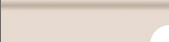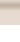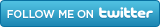HomeAll Speed TipsOther TipsOptimization SoftwareTech NewsInternet SecurityComputer JargonSubscribe via EmailRecent Articles
Learn To Repair PCs With The Right Course

How to Optimize Droid Razr Battery

# Converting Units in Windows 7

One nice upgrade to Windows 7 is the unit converter on the built-in calculator program. This can be a very handy tool if you are someone who needs to do this often. Just follow the simple steps below to convert units of power, mass, time, and volume.

1. Click the Start button, select "All Programs", "Accessories", then "Calculator" to open up the Windows 7 Calculator.

2. Next, select the "View" menu then click on "Unit Conversion".

3. A unit conversion windows will appear to the right of the calculator. Next, you can click the pull-down and select the unit type that you want to convert.

4. In the "From" box, type in the value of the starting unit.

5. At the very bottom of the Unit conversion panel, you will see a "TO" box. From the drop-down, select the unit you want to convert to.

At this point you will see the converted value right below the TO header. One nice thing is that you can copy this value using edit->copy to bring the value to another document, excel sheet, etc.

• Degree

## Area

• Acres
• Hectares
• Square centimeter
• Square feet
• Square inch
• Square kilometer
• Square meters
• Square mile
• Square millimeter
• Square yard

## Energy

• British Thermal Unit
• Calorie
• Electron-volts
• Foot-pound
• Joule
• Kilocalorie
• Kilojoule

## Length

• Angstrom
• Centimeters
• Chain
• Fathom
• Feet
• Hand
• Inch
• Kilometers
• Meter
• Microns
• Mile
• Millimeters
• Nanometers
• Nautical miles
• PICA
• Rods
• Span
• Yard

## Power

• BTU/minute
• Foot-pound/minute
• Horsepower
• Kilowatt
• Watt

## Pressure

• Atmosphere
• Bar
• Kilo Pascal
• Millimeter of mercury
• Pascal
• Pound per square inch (PSI)

## Temperature

• Degrees Celsius
• Degrees Fahrenheit
• Kelvin

• Day
• Hour
• Microsecond
• Millisecond
• Minute
• Second
• Week

## Velocity

• Centimeter per second
• Feet per second
• Kilometer per hour
• Knots
• Mach (at std. atm)
• Meter per second
• Miles per hour

## Volume

• Cubic centimeter
• Cubic feet
• Cubic inch
• Cubic meter
• Cubic yard
• Fluid ounce (UK)
• Fluid ounce (US)
• Gallon (UK)
• Gallon (US)
• Liter
• Pint (UK)
• Pint (US)
• Quart (UK)
• Quart (US)

## Weight Or Mass

• Carat
• Centigram
• Decigram
• Dekagram
• Gram
• Hectogram
• Kilogram
• Long ton
• Milligram
• Ounce
• Pound
• Short ton
• Stone
• Tonne

 If you enjoyed this post, please Tweet or
-By
TAGS: Windows 7 Tips